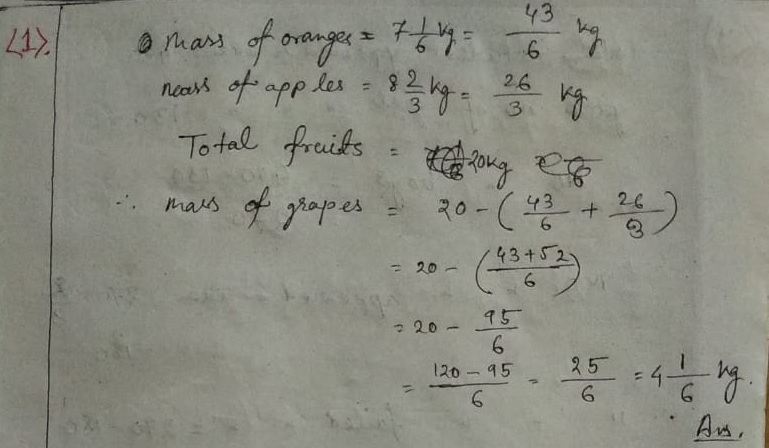# ML Aggarwal CBSE Solutions Class 8 Math First Chapter Rational Numbers Exercise 1.6

### ML Aggarwal CBSE Solutions Class 8 Math 1st Chapter Rational Numbers Exercise 1.6(2) Population of city = 6,63,432

No of adult males = 6,63,432/2

= 3,31716

No of adult females = 6,63,432/3

= 2,21,144

∴ No of children = 6,63,432 – (3,31,716 + 2,21,144)

= 6,62,432 – 5,52,860

= 1,10,572

(3) Total student appeared in exam = 400

No of girls appeared in exam = 130

∴ No of boys = 400 – 130

= 270

∴No of boys appeared in exam = 270 x 2/3

= 180

∴ No of boys failes in the exam = 270 – 180

= 90(9) Total income = Rs. 40,000 per month

∴ food expanses = 40,000 x 3/8

= Rs. 15,000

∴ rest money = 40,000 – 15,000

= Rs. 25,000

LIC Premium expanses = 25000 x 1/5

= Rs. 5,000

rest money = 25,000 – 5000 = Rs. 20,000

∴ Other expanses 20,000 x 1/2 = Rs. 10,000

∴ rest money = 20,000 – 10,000 = Rs. 10,000(12) Let, Nothers contribution = x

elder son’s contribution = x X 3/8

= 3x/8

younger son’s contribution = x X 1/2

= x/2

∴ According to question

3x/8 + x/2 + x = 60,000

Or, 3x+4x+8x/8 = 60,000

Or, 15x = 60,000 x 8

Or, x = 60000×8/15

= 32,000

Mothers contribution = Rs. 32,000

elder son’s contribution = 32,000 x 3/8

= Rs. 12,000

younger sons contribution = 32,000 x 1/2

= Rs. 16,000

(13) Total Students = 56

number of girls = x

∴ number of boys = 2x/5

∴ According to the question

x + 2x/5 = 56

Or, 5x+2x/5 = 56

Or, 7x = 56 x 5

Or, x = 56×5/7

= 40

∴ number of girls = 40

number of boys 40 x 2/5

= 16

(14) Let, total money = x

∴ donation of school = x x 1/10 = x/10

rest of money = x – x/10

= 10x-x/10

9x/10

donation of church = 1/6 x 9x/10

3x/20

rest of money = 9x/10 – 3x/20

= 18x-3x/20

15x/20

According to the question

15x/20 ÷ 3 = 50000

(15) Let, the number is = x

According to question

(x X 1/4) + (x X 1/3) = x/2 + 15

Or, x/4 + x/3 = x+30/2

Or, 3x+4x/12 = x+30/2

Or, 7x/6 = x+30

Or, 7x = 6x + 180

Or, 7x – 6x = 1800

Or, x = 180

(16) Let, the given number is = x

According to the question

(5/4 X x) – (4/5 X x) = 36

Or, 5x/4 – 4x/5 = 36

Or, 25x-16x/20 = 36

Or, 9x/20 = 36

Or, x = 36×20/9

= 80

Updated: February 15, 2021 — 9:04 pm